2 . 含参数广义积分

[一致收敛性]设函数f(x,y)是定义在区域R(ax<, y1<y<y2)上的连续函数，若对任意给定的ε>0，都存在只与ε有关的正数B=B(ε),使得当bB时，对区间(y1y2)内一切y不等式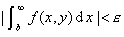[一致收敛判别法]

1°柯西判别积分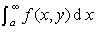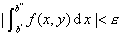2°外尔斯特拉斯判别法  设函数f(x,y)(x的函数)在任一有限区间[a,A]上可积，若存在与参数y无关的函数F(x)，它在区间[a,)上可积，并且对于区间(y1y2)内的一切y

|f(x,y)|F(x)               (xa)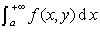[对参数的微分法](i)函数f(x,y)在区域R(ax<,y1<y<y2)内连续，并对参数y可微，(ii)积分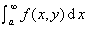收敛，(iii)积分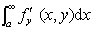在区间(y1y2)内一致收敛,则当y1<y<y2时，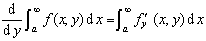[对参数的积分法]若函数f (x,y)在区域R(ax<, y1<y<y2)内连续 ，并且在区间(y1y2)内一致收敛，则